# Numpy standard deviation explained

This tutorial will explain how to use the Numpy standard deviation function (AKA, np.std).

At a high level, the Numpy standard deviation function is simple. It calculates the standard deviation of the values in a Numpy array.

But the details of exactly how the function works are a little complex and require some explanation.

That being said, this tutorial will explain how to use the Numpy standard deviation function.

It will explain the syntax of np.std(), and show you clear, step-by-step examples of how the function works.

The tutorial is organized into sections. You can click on any of the following links, which will take you to the appropriate section.

Having said that, if you’re relatively new to Numpy, you might want to read the whole tutorial.

## A quick review of Numpy

Let’s just start off with a veeeery quick review of Numpy.

What is Numpy?

### Numpy is a toolkit for working with numeric data

To put it simply, Numpy is a toolkit for working with numeric data.

First, Numpy has a set of tools for creating a data structure called a Numpy array.

You can think of a Numpy array as a row-and-column grid of numbers. Numpy arrays can be 1-dimensional, 2-dimensional, or even n-dimensional.

A 2D array looks something like this:For simplicity sake, in this tutorial, we’ll stick to 1 or 2-dimentional arrays.

There are a variety of ways to create different types of arrays with different kinds of numbers. A few other tools for creating Numpy arrays include numpy arrange, numpy zeros, numpy ones, numpy tile, and other methods.

Regardless of how you create your Numpy array, at a high level, they are simply arrays of numbers.

### Numpy provides tools for manipulating Numpy arrays

Numpy not only provides tools for creating Numpy arrays, Numpy also provides tools for working with Numpy arrays.

Some of the most important of these Numpy tools are Numpy functions for performing calculations.

There’s a whole set of Numpy functions for doing things like:

… and a variety of other computations.

The Numpy standard deviation is essentially a lot like these other Numpy tools. It is just used to perform a computation (the standard deviation) of a group of numbers in a Numpy array.

## A quick introduction to Numpy standard deviation

At a very high level, standard deviation is a measure of the spread of a dataset. In particular, it is a measure of how far the datapoints are from the mean of the data.

Let’s briefly review the basic calculation.

Standard deviation is calculated as the square root of the variance.

So if we have a dataset with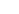numbers, the variance will be:

(1)And the standard deviation will just be the square root of the variance:

(2)Where: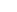= the individual values in the dataset= the number of values in the dataset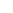= the mean of the valuesMost of the time, calculating standard deviation by hand is a little challenging, because you need to compute the mean, the deviations of each datapoint from the mean, then the square of the deviations, etc. Frankly, it’s a little tedious.

However, if you’re working in Python, you can use the Numpy standard deviation function to perform the calculation for you.

##### A quick note if you’re new to statistics

Because this blog post is about using the numpy.std() function, I don’t want to get too deep into the weeds about how the calculation is performed by hand. This tutorial is really about how we use the function. So, if you need a quick review of what standard deviation is, you can watch this video.

Ok. Having quickly reviewed what standard deviation is, let’s look at the syntax for np.std.

## The syntax of np.std

The syntax of the Numpy standard deviation function is fairly simple.

I’ll explain it in just a second, but first, I want to tell you one quick note about Numpy syntax.

#### A quick note: the exact syntax depends on how you import Numpy

Typically, when we write Numpy syntax, we use the alias “np”. That’s the common convention among most data scientists.

To set that alias, you need to import Numpy like this:

import numpy as np


If we import Numpy with this alias, we’ll can call the Numpy standard deviation function as np.std().

Ok, that being said, let’s take a closer look at the syntax.

### np.std syntax

At a high level, the syntax for np.std looks something like this:As I mentioned earlier, assuming that we’ve imported Numpy with the alias “np” we call the function with the syntax np.std().

Then inside of the parenthesis, there are several parameters that allow you to control exactly how the function works.

Let’s take a look at those parameters.

### The parameters of numpy.std

There are a few important parameters you should know:

• a
• axis
• dtype
• ddof
• keepdims
• out

Let’s take a look at each of them.

###### a(required)

The a parameter specifies the array of values over which you want to calculate the standard deviation.

Said differently, this enables you to specify the input array to the function.

Appropriate inputs include Numpy arrays, but also “array like” objects such as Python lists.

Importantly, you must provide an input to this parameter. An input is required.

Having said that, the parameter itself can be implicit or explicit. What I mean by that, is that you can directly type the parameter a=, OR you can leave the parameter out of your syntax, and just type the name of your input array.

I’ll show you examples of this in example 1.

###### axis

The axis parameter enables you to specify an axis along which the standard deviation will be computed.

To understand this, you really need to understand axes.

Numpy arrays have axes.

You can think of an “axis” like a direction along the array.

In a 2-dimensional array, there will be 2 axes: axis-0 and axis-1.

In a 2D array, axis-0 points downward along the rows, and axis-1 points horizontally along the columns.

Visually, you can visualize the axes of a 2D array like this:Using the axis parameter, you can compute the standard deviation in a particular direction along the array.

This is best illustrated with examples, so I’ll show you an example in example 2.

(For a full explanation of Numpy array axes, see our tutorial called Numpy axes explained.)

###### dtype

(optional)
The dtype parameter enables you to specify the data type that you want to use when np.std computes the standard deviation.

If the data in the input array are integers, then this will default to float64.

Otherwise, if the data in the input array are floats, then this will default to the same float type as the input array.

###### ddof

(optional)
This enables you to specify the “degrees of freedom” for the calculation.

To understand this, you need to look at equation 2 again.In this equation, the first term is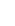.

Remember:is the number of values in the array or dataset.

But if we’re thinking in statistical terms, there’s actually a difference between computing a population standard deviation vs a sample standard deviation.

If we compute a population standard deviation, we use the termin our equation.

However, when we compute the standard deviation on a sample of data (a sample of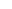datapoints), then we need to modify the equation so that the leading term is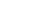. In that case, the equation for a sample standard deviation becomes:

(3)How do we implement this with np.std?

We can do this with the ddof parameter, by setting ddof = 1.

And in fact, we can set the ddof term more generally. When we use ddof, it will modify the standard deviation calculation to become:

(4)###### out

(optional)
The out parameter enables you to specify an alternative array in which to put the output.

It should have the same shape as the expected output.

###### keepdims

(optional)
The keepdims parameter can be used to “keep” the original number of dimensions. When you set keepdims = True, the output will have the same number of dimensions as the input.

Remember: when we compute the standard deviation, the computation will “collapse” the number of dimensions.

For example, if we input a 2-dimensional array as an input, then by default, np.std will output a number. A scalar value.

But if we want the output to be a number within a 2D array (i.e., an output array with the same dimensions as the input), then we can set keepdims = True.

To be honest, some of these parameters are a little abstract, and I think they will make a lot more sense with examples.

Let’s take a look at some examples.

## Examples of how to use Numpy standard deviation

Here, we’ll work through a few examples. We’ll start simple and then increase the complexity.

Examples:

#### Run this code first

Before you run any of the example code, you need to import Numpy.

To do this, you can run the following code:

import numpy as np


This will import Numpy with the alias “np“.

### EXAMPLE 1: Calculate standard deviation of a 1 dimensional array

Here, we’ll start simple.

We’re going to calculate the standard deviation of 1-dimensional Numpy array.

###### Create 1D array

First, we’ll just create our 1D array:

array_1d = np.array([12, 14, 99, 72, 42, 55])

###### Calculate standard dev

Now, we’ll calculate the standard deviation of those numbers.

np.std(array_1d)


OUT:

30.84369195367723


So what happened here?

The np.std function just computed the standard deviation of the numbers [12, 14, 99, 72, 42, 55] using equation 2 that we saw earlier. Each number is one of thein that equation.

###### One quick note

In the above example, we did not explicitly use the a= parameter. That is because np.std understands that when we provide an argument to the function like in the code np.std(array_1d), the input should be passed to the a parameter.

Alternatively, you can also explicitly use the a= parameter:

np.std(a = array_1d)


OUT:

30.84369195367723


### EXAMPLE 2: Calculate the standard deviation of a 2-dimensional array

Ok. Now, let’s look at an example with a 2-dimensional array.

###### Create 2-dimensional array

Here, we’re going to create a 2D array, using the np.random.randint function.

np.random.seed(22)
array_2d = np.random.randint(20, size =(3, 4))


This array has 3 rows and 4 columns.

Let’s print it out, so we can see it.

print(array_2d)


OUT:

[[ 4 12  0  4]
[ 6 11  8  4]
[18 14 13  7]]


This is just a 2D array that contains 12 random integers between 0 and 20.

###### Compute standard deviation with np.std

Okay, let’s compute the standard deviation.

np.std(array_2d)


OUT:

5.007633062524539


Here, numpy.std() is just computing the standard deviation of all 12 integers.

The standard deviation is 5.007633062524539.

### EXAMPLE 3: Compute the standard deviation of the columns

Now, we’re going to compute the standard deviation of the columns.

To do this, we need to use the axis parameter. (You learned about the axis parameter in the section about the parameters of numpy.std)

Specifically, we need to set axis = 0.

Why?

As I mentioned in the explanation of the axis parameter earlier, Numpy arrays have axes.

In a two dimensional array, axis-0 is the axis that points downwards.When we use numpy.std with axis = 0, that will compute the standard deviations downward in the axis-0 direction.

Let’s take a look at an example so you can see what I mean.

##### Create 2-dimensional array

First, we’ll create a 2D array, using the np.random.randint function.

(This is the same array that we created in example 2, so if you already created it, you shouldn’t need to create it again.)

np.random.seed(22)
array_2d = np.random.randint(20, size =(3, 4))


Let’s print it out, so we can see it.

print(array_2d)


OUT:

[[ 4 12  0  4]
[ 6 11  8  4]
[18 14 13  7]]


This is just a 2D array that contains integers between 0 and 20.

###### Use np.std to compute standard deviation of the columns

Now, we’ll set axis = 0 inside of np.std to compute the standard deviations of the columns.

np.std(array_2d, axis = 0)


OUT:

array([6.18241233, 1.24721913, 5.35412613, 1.41421356])

###### Explanation

What’s going on here?

When we use np.std with axis = 0, Numpy will compute the standard deviation downward in the axis-0 direction. Remember, as I mentioned above, axis-0 points downward.

This has the effect of computing the standard deviation of each column of the Numpy array.Now, let’s do a similar example with the row standard deviations.

### EXAMPLE 4: Use np.std to compute the standard deviations of the rows

Now, we’re going to use np.std to compute the standard deviations horizontally along a 2D numpy array.

Remember what I said earlier: numpy arrays have axes. The axes are like directions along the Numpy array. In a 2D array, axis-1 points horizontally, like this:So, if we want to compute the standard deviations horizontally, we can set axis = 1. This has the effect of computing the row standard deviations.

Let’s take a look.

##### Create 2-dimensional array

To run this example, we’ll again need a 2D Numpy array, so we’ll create a 2D array using the np.random.randint function.

(This is the same array that we created in example 2, so if you already created it, you shouldn’t need to create it again.)

np.random.seed(22)
array_2d = np.random.randint(20, size =(3, 4))


Let’s print it out, so we can see it.

print(array_2d)


OUT:

[[ 4 12  0  4]
[ 6 11  8  4]
[18 14 13  7]]


This is just a 2D array that contains integers between 0 and 20.

###### Use np.std to compute standard deviation of the rows

Now, we’ll use np.std with axis = 1 to compute the standard deviations of the rows.

np.std(array_2d, axis = 1)


OUT:

array([4.35889894, 2.58602011, 3.93700394])

###### Explanation

If you understood example 3, this new example should make sense.

When we use np.std and set axis = 1, Numpy will compute the standard deviations horizontally along axis-1.Effectively, when we use Numpy standard deviation with axis = 1, the function computes the standard deviation of the rows.

### EXAMPLE 5: Change the degrees of freedom

Now, let’s change the degrees of freedom.

Here in this example, we’re going to create a large array of numbers, take a sample from that array, and compute the standard deviation on that sample.

First, let’s create our arrays.

##### Create Numpy array

First, we’ll just create a normally distributed Numpy array with a mean of 0 and a standard deviation of 10.

To do this, we’ll use the Numpy random normal function. Note that we’re using the Numpy random seed function to set the seed for the random number generator. For more information on this, read our tutorial about np.random.seed.

np.random.seed(22)
population_array = np.random.normal(size = 100, loc = 0, scale = 10)


Ok. Now we have a Numpy array, population_array, that has 100 elements that have a mean of 0 and a standard deviation of 10.

##### Create sample

Now, we’ll use Numpy random choice to take a random sample from the Numpy array, population_array.

np.random.seed(22)
sample_array = np.random.choice(population_array, size = 10)


This new array, sample_array, is a random sample of 10 elements from population_array.

We’ll use sample_array when we calculate our standard deviation using the ddof parameter.

##### Calculate the standard deviation of the sample

Now, we’ll calculate the standard deviation of the sample.

Specifically, we’re going to use the Numpy standard deviation function with the ddof parameter set to ddof = 1.

np.std(sample_array, ddof = 1)


OUT:

10.703405562234051

##### Explanation

Here, we’ve calculated:And when we set ddof = 1, the equation evaluates to:To be clear, when you calculate the standard deviation of a sample, you will set ddof = 1.

To be honest, the details about why are a little technical (and beyond the scope of this post), so for more information about calculating a sample standard deviation, I recommend that you watch this video.

Keep in mind, that for some other instances, you can set ddof to other values besides 1 or 0. If you don’t use the ddof parameter at all, it will default to 0.

No matter what value you select, the Numpy standard deviation function will compute the standard deviation with the equation:### EXAMPLE 6: Use the keepdims parameter in np.std

Ok. Finally, we’ll do one last example.

Here, we’re going to set the keepdims parameter to keepdims = True.

##### Create 2-dimensional array

First, we’ll create a 2D array, using the np.random.randint function.

(This is the same array that we created in example 2, so if you already created it, you shouldn’t need to create it again.)

np.random.seed(22)
array_2d = np.random.randint(20, size =(3, 4))


Let’s print it out:

print(array_2d)


OUT:

[[ 4 12  0  4]
[ 6 11  8  4]
[18 14 13  7]]

###### Check the dimensions

Now, let’s take a look at the dimensions of this array.

array_2d.ndim


OUT:

2


This is a 2D array, just like we intended.

###### Compute the standard deviation, and check the dimensions

Ok. Now, we’re going to compute the standard deviation, and check the dimensions of the output.

output = np.std(array_2d)


Let’s quickly print the output:

print(output)


OUT:

5.007633062524539


So the standard deviation is 5.007633062524539.

Now, what’s the dimensions of the output?

output.ndim


OUT:

0


The output has 0 dimensions (it’s a scalar value).

Why?

When np.std computes the standard deviation, it’s computing a summary statistic. In this case, the function is taking a large number of values and collapsing them down to a single metric.

So the input was 2-dimensional, but the output is 0-dimensional.

What if we want to change that?

What if we want the output to technically have 2-dimensions?

We can do that with the keepdims parameter.

#### Keep the original dimensions when we use np.std

Here, we’ll set keepdims = True to make the output the same dimensions as the input.

output_2d = np.std(array_2d, keepdims = True)


Now, let’s look at the output:

print(output_2d)


OUT:

[[5.00763306]]


Notice that the output, the standard deviation, is still 5.00763306. But the result is enclosed inside of double brackets.

Let’s inspect output_2d and take a closer look.

type(output_2d)


OUT:

numpy.ndarray


So, output_2d is a Numpy array, not a scalar value.

Let’s check the dimensions:

output_2d.ndim


OUT:

2


This Numpy array, output_2d, has 2 dimensions.

This is the same number of dimensions as the input.

What happened?

When we set keepdims = True, that caused the np.std function to produce an output with the same number of dimensions as the input. Even though there are not any rows and columns in the output, the output output_2d has 2 dimensions.

So, in case you ever need your output to have the same number of dimensions as your input, you can set keepdims = True.

(This also works when you use the axis parameter … try it!)

Now that you’ve learned about Numpy standard deviation and seen some examples, let’s review some frequently asked questions about np.std.

### Question 1: Why does numpy std() give a different result than matlab std() or another programing language?

The simple reason is that matlab calculates the standard dev according to the following:(Many other tools use the same equation.)

However, Numpy calculates with the following:Notice the subtle difference between thevs the.

To fix this, you can use the ddof parameter in Numpy.

If you use np.std with the ddof parameter set to ddof = 1, you should get the same answer as matlab.

Do you have other questions about the Numpy standard deviation function?

The examples you’ve seen in this tutorial should be enough to get you started, but if you’re serious about learning Numpy, you should enroll in our premium course called Numpy Mastery.

There’s a lot more to learn about Numpy, and Numpy Mastery will teach you everything, including:

• How to create Numpy arrays
• How to use the Numpy random functions
• What the “Numpy random seed” function does
• How to reshape, split, and combine your Numpy arrays
• and more …

Moreover, it will help you completely master the syntax within a few weeks. You’ll discover how to become “fluent” in writing Numpy code.

Find out more here:

Joshua Ebner

Joshua Ebner is the founder, CEO, and Chief Data Scientist of Sharp Sight. Prior to founding the company, Josh worked as a Data Scientist at Apple. He has a degree in Physics from Cornell University.

### 4 thoughts on “Numpy standard deviation explained”

1. You’re a great guy.

Well done.